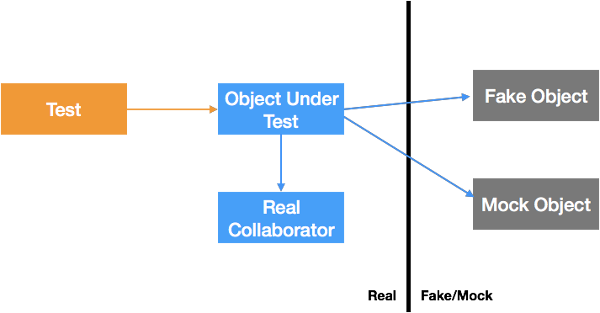When testing tricky collaborators, we may create fake or mock objects to make life easier.

In effect, we draw a line: on one side, real objects participate; on the other, it’s fakes and/or mocks.## Consequences of the Line

A common strategy for testing with mocks:

• Test standalone objects directly

• Use fakes and mocks to test objects with tricky collaborators

• Don’t test objects owned by someone else

• Don’t “unit” test some objects at all; test them with with system or acceptance tests.

What objects have you left untested in your app?

One case I commonly see: database access is left untested. When the data class is just mapping simple fields, the risk is low. But sometimes, the data requires complicated searches or queries. (I recall one that was multiple pages long, touching up to a dozen tables. Unit-testing this would have required dozens, maybe even 100+, tests, so it was never tested.)

## A Tiny Case Study

We recently developed an exercise where you could practice injecting a fake for testing purposes.

The idea is that you’re generating a character in Dungeons & Dragons. To determine the character’s strength, you roll four six-sided dice, and use the sum of the biggest three rolls as your score.

Here’s the code for the Maker:

To test this, you’d like to test various combinations of high and low dice, to make sure you can generate proper character strengths. Since the Dice class is based around a random number generator, it’s handy to create a fake.

With the fake in place, you can test the Maker code, and be sure that it properly chooses and sums the dice.

We drew a line with Maker and Dice, and left Dice on the “fake” side of the line. In fact, we haven’t tested the Dice class at all. Take a peek at it:

We see something interesting right away - this method takes an (optional) argument and can generate a sequence of rolls. (And our Maker code didn’t use that feature.) Also, it’s recursive - the case with an argument calls the same method without one.

Perhaps that’s a missed opportunity; perhaps it’s unnecessary complexity.

I’m more interested in the output values. We’re supposed to get random numbers 1 to 6: is that what we get?

Well, `Math.random()` produces a number x such that 0 <= x < 1, a “half-open interval” [0, 1).

Suppose it generates a 0, then Math.round(0 * 5 + 1) = Math.round(0 + 1) = Math.round(1) = 1.

Suppose it generates a .9999, then Math.round(.9999 * 5 + 1) = Math.round(4.9995 + 1) = Math.round(5.9995) = 6.

Intermediate numbers will provide other values in the 1 to 6 range. Sounds good, right?

## The Missed Assumption

There’s a hidden assumption: the numbers should be equally likely, just like legal dice.

Do we meet that? Assume that `Math.random()` works as advertised, generating equally likely numbers in that [0, 1) range.

``````    Math.random() is [0, 1)
Math.random() * 5 is [0, 5)
Math.random() * 5 + 1 is [1, 6)
``````

For analyzing round(), it’s easiest to divide this up into intervals 0.5 wide:

``````    round( [1, 1.5) ) => 1
round( [1.5, 2) ) => 2
round( [2, 2.5) ) => 2
round( [2.5, 3) ) => 3
round( [3, 3.5) ) => 3
round( [3.5, 4) ) => 4
round( [4, 4.5) ) => 4
round( [4.5, 5) ) => 5
round( [5, 5.5) ) => 5
round( [5.5, 6) ) => 6
``````

That is bad news: 1 and 6 are only half as likely to appear as 2 through 5. If you use this to generate characters, they will tend to be weighted toward the middle values. If I did my math right, you should expect the worst score 1 in 1296 times but with this code it’s only 1 in 10,000.

What’s the fix? There are lots of possibilities; let’s use a variant that uses `Math.trunc()` instead of `Math.round()`: `Math.trunc(6 * Math.random()) + 1`

## How Did This Happen?

The original code was in Java. In Java, there’s a random function that returns integers in a range.

The code was translated to JavaScript. Since JavaScript only has a random number returning [0, 1) values, the programmer wrote a conversion formula in the Dice class.

The original code tested the Maker class well, but neglected the Dice class. The error was spotted by a chance inspection; it would have been hard to catch without a test that specifically checked the distribution of values.

## Lessons

• Testing with random numbers is tricky. (For example, there are other properties we want, such as no sequential dependency between numbers; we’re relying on `Math.random()` to handle this.)

• When you test with fakes and mocks, you’re drawing a line. If you never test the real object (but only use fakes or mocks in testing other objects), you don’t know if the parts you faked work properly.

• An integration test may cover the real objects that were faked out, but even there we might miss issues.

• This isn’t a warning against fakes and mocks, but rather a reminder that unit tests can’t catch everything, especially for code they don’t test.

## Acknowledgments

Thanks to Alex Freire, Tim Ottinger, David Nunn, Amr Elssamadisy, and Joshua Kerievsky for comments on an earlier draft.